Hostname: page-component-7d684dbfc8-2bg86 Total loading time: 0.001 Render date: 2023-09-27T13:43:35.243Z Has data issue: false Feature Flags: { "corePageComponentGetUserInfoFromSharedSession": true, "coreDisableEcommerce": false, "coreDisableSocialShare": false, "coreDisableEcommerceForArticlePurchase": false, "coreDisableEcommerceForBookPurchase": false, "coreDisableEcommerceForElementPurchase": false, "coreUseNewShare": true, "useRatesEcommerce": true } hasContentIssue false

# Asymptotic Improvements of Lower Bounds for the Least Common Multiples of Arithmetic Progressions

Published online by Cambridge University Press:  20 November 2018

## Abstract

Core share and HTML view are not possible as this article does not have html content. However, as you have access to this content, a full PDF is available via the ‘Save PDF’ action button.

For relatively prime positive integers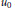${{u}_{0}}$ and$r$, we consider the least common multiple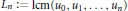${{L}_{n}}\,:=\,\text{lcm}\left( {{u}_{0}},\,{{u}_{1}},\,.\,.\,.\,,\,{{u}_{n}} \right)$ of the finite arithmetic progression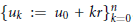$\left\{ {{u}_{k}}\,:=\,{{u}_{0}}\,+\,kr \right\}_{k=0}^{n}$. We derive new lower bounds on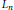${{L}_{n}}$ that improve upon those obtained previously when either${{u}_{0}}$ or$n$ is large. When$r$ is prime, our best bound is sharp up to a factor of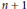$n\,+\,1$ for${{u}_{0}}$ properly chosen, and is also nearly sharp as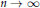$n\,\to \,\infty$.

## Keywords

Type
Research Article
Information
Canadian Mathematical Bulletin , 01 September 2014 , pp. 551 - 561

## References

[BKS02] Bateman, P., Kalb, J., and Stenger, A., A limit involving least common multiples: 10797. Amer. Math. Monthly 109 (2002), no. 4, 393394. http://dx.doi.org/10.2307/2695513 CrossRefGoogle Scholar
[Far05] Farhi, B., Minorations non triviales du plus petit commun multiple de certaines suites finies d’entiers. C. R. Math. Acad. Sci. Paris 341 (2005), no. 8, 469474. http://dx.doi.org/10.1016/j.crma.2005.09.019 CrossRefGoogle Scholar
[Far07] Farhi, B., Nontrivial lower bounds for the least common multiple of some finite sequences of integers. J. Number Theory 125 (2007), no. 2, 393411. http://dx.doi.org/10.1016/j.jnt.2006.10.017 CrossRefGoogle Scholar
[FK09] Farhi, B. and Kane, D., New results on the least common multiple of consecutive integers. Proc. Amer. Math. Soc. 137 (2009), no. 6, 19331939. http://dx.doi.org/10.1090/S0002-9939-08-09730-X CrossRefGoogle Scholar
[Han72] Hanson, D., On the product of the primes. Canad. Math. Bull. 15 (1972), 3337. http://dx.doi.org/10.4153/CMB-1972-007-7 CrossRefGoogle Scholar
[HF06] Hong, S. and Feng, W., Lower bounds for the least common multiple of finite arithmetic progressions. C. R. Acad. Sci. Paris 343 (2006), no. 1112, 695698. http://dx.doi.org/10.1016/j.crma.2006.11.002 CrossRefGoogle Scholar
[HK10] Hong, S. and Kominers, S. D., Further improvements of lower bounds for the least common multiples of arithmetic progressions. Proc. Amer. Math. Soc. 138 (2010), no. 3, 809813. http://dx.doi.org/10.1090/S0002-9939-09-10083-7 CrossRefGoogle Scholar
[HQ11] Hong, S. and Qian, G., The least common multiple of consecutive arithmetic progression terms. Proc. Edinb. Math. Soc. (2) 54 (2011), no. 2, 431441. http://dx.doi.org/10.1017/S0013091509000431 CrossRefGoogle Scholar
[HY08] Hong, S. and Yang, Y., Improvements of lower bounds for the least common multiple of finite arithmetic progressions. Proc. Amer. Math. Soc. 136 (2008), no. 12, 41114114. http://dx.doi.org/10.1090/S0002-9939-08-09565-8 CrossRefGoogle Scholar
[IK04] Iwaniec, H. and Kowalski, E., Analytic number theory. American Mathematical Society Colloquium Publications, 53, American Mathematical Society, Providence, RI, 2004.Google Scholar
[Nai82] Nair, M., On Chebyshev-type inequalities for primes. Amer. Math. Monthly 89 (1982), no. 2, 126129. http://dx.doi.org/10.2307/2320934 CrossRefGoogle Scholar
[WTH13] Wu, R., Tan, Q., and Hong, S., New lower bounds for the least common multiples of arithmetic progressions. Chin. Ann. Math. Ser. B 34 (2013), no. 6, 861864. http://dx.doi.org/10.1007/s11401-013-0805-9 CrossRefGoogle Scholar